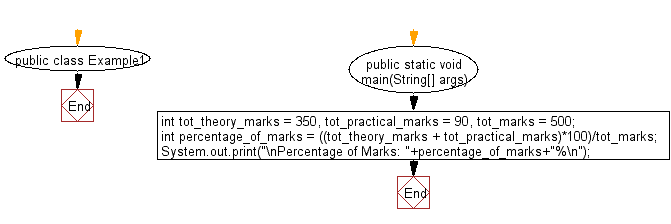﻿ Java Math Exercises: Round up the result of integer division - w3resource# Java Math Exercises: Round up the result of integer division

## Java Math Exercises: Exercise-1 with Solution

Write a Java program to round up the result of integer division.

Sample Solution:

Java Code:

``````import java.util.*;
public class Example1 {
public static void main(String[] args) {
int tot_theory_marks = 350, tot_practical_marks = 90, tot_marks = 500;
int percentage_of_marks = ((tot_theory_marks + tot_practical_marks)*100)/tot_marks;
System.out.print("\nPercentage of Marks: "+percentage_of_marks+"%\n");
}
}
``````

Sample Output:

```Percentage of Marks: 88%
```

Flowchart:Java Code Editor:

What is the difficulty level of this exercise?

﻿

## Java: Tips of the Day

Parsing dates:

```import java.io.*;
import java.util.*;
import java.text.*;

String s = "2001/09/23 14:39";

SimpleDateFormat formatter = new SimpleDateFormat ("yyyy/MM/dd H:mm");
Date d = formatter.parse(s, new ParsePosition(0));
```